Related Articles
Java Program to Implement Binomial Tree
• Last Updated : 22 Feb, 2021

A binomial tree is a personification of the built-in values, which an option may take at different intervals of time period.

At any node, the value of the option depends on the price of the fundamental asset in the probability that either price will decrease or increase at any given node.

Implementation of Binomial Tree :

## Java

 `// Java Program to Implement Binomial Tree`` ` `import` `java.util.Scanner;`` ` `public` `class` `BinomialTreeTest {``    ``public` `static` `void` `main(String[] args)``    ``{``        ``Scanner sc = ``new` `Scanner(System.in);`` ` `        ``System.out.println(``"*** BINOMIAL TREE IMPLEMENTATION ***"``);``       ` `        ``// Input for order of binomial tree``        ``System.out.println(``"Enter the order of binomial tree"``);``        ``int` `order = sc.nextInt();`` ` `        ``// Creating the object of binary tree``        ``BinomialTreeExample tree = ``new` `BinomialTreeExample(order);`` ` `        ``// variable to toggle condition of loop``        ``boolean` `exit = ``false``;`` ` `        ``// while loop``        ``while` `(!exit) {``           ` `            ``// menu for the user``            ``System.out.println(``"\nBinomial Tree Operations\n"``);``            ``System.out.println(``"1. Insert element in tree "``);``            ``System.out.println(``"2. Find size of tree"``);``            ``System.out.println(``"3. check whether tree is empty or not"``);``            ``System.out.println(``"4. Clear the tree"``);``            ``System.out.println(``"5. Print the tree"``);``            ``System.out.println(``"6. Exit"``);`` ` `            ``// taking choice of user``            ``int` `choice = sc.nextInt();``           ` `            ``switch` `(choice) {``            ``case` `1``:``                ``System.out.println(``"Enter element to insert"``);``                ``tree.insert(sc.nextInt());``                ``break``;`` ` `            ``case` `2``:``                ``System.out.println(``"Size of tree is = "``                                   ``+ tree.getSize());``                ``break``;`` ` `            ``case` `3``:``                ``System.out.println(``"Is tree empty? "``                                   ``+ tree.isEmpty());``                ``break``;`` ` `            ``case` `4``:``                ``tree.clear();``                ``System.out.println(``"Tree is Cleared successfully.."``);``                ``break``;``            ``case` `5``:``                ``System.out.println(``"Elements of the tree are : "``);``                ``tree.printTree();``                ``break``;`` ` `            ``case` `6``:``                ``System.out.println(``"Exited successfully.."``);``                ``exit = ``true``;``                ``break``;``            ``}``        ``}``        ``sc.close();``    ``}``}`` ` `class` `BinomialTreeExample {``   ` `    ``// class binomial tree node``   ` `    ``class` `BinoTreeNode {``        ``int` `data;``        ``int` `numNodes;``        ``BinoTreeNode arr[];`` ` `        ``// constructor of binomial tree node``       ` `        ``public` `BinoTreeNode(``int` `n)``        ``{``            ``// initializing the values``            ``data = -``1``;``           ` `            ``numNodes = n;``            ``arr = ``new` `BinoTreeNode[numNodes];``        ``}``    ``}`` ` `    ``private` `BinoTreeNode root;``    ``private` `int` `order, size;`` ` `    ``// constructor of binomial tree example``    ``public` `BinomialTreeExample(``int` `n)``    ``{``        ``size = ``0``;``        ``order = n;``        ``root = ``new` `BinoTreeNode(order);``        ``createTree(root);``    ``}`` ` `    ``// method to create a tree``    ``private` `void` `createTree(BinoTreeNode bt)``    ``{``        ``int` `n = bt.numNodes;``        ``if` `(n == ``0``)``            ``return``;``       ` `        ``for` `(``int` `i = ``0``; i < n; i++)``        ``{``            ``bt.arr[i] = ``new` `BinoTreeNode(i);``            ``createTree(bt.arr[i]);``        ``}``    ``}`` ` `    ``// method to clear tree``    ``public` `void` `clear()``    ``{``        ``size = ``0``;``        ``root = ``new` `BinoTreeNode(order);``        ``createTree(root);``    ``}`` ` `    ``// method to check tree is empty or not``    ``public` `boolean` `isEmpty() { ``return` `size == ``0``; }`` ` `    ``// method to get the size of tree``    ``public` `int` `getSize() { ``return` `size; }`` ` `    ``// public method to insert an element in tree which is``    ``// called by tester class publically``    ``public` `void` `insert(``int` `val)``    ``{``        ``try` `{``            ``insert(root, val);``        ``}``        ``catch` `(Exception e) {``            ``System.out.println(``"Inserted successfully.."``);``        ``}``    ``}`` ` `    ``// private method to insert a tree which is called``    ``// internally by method insert``    ``private` `void` `insert(BinoTreeNode bt, ``int` `val)``        ``throws` `Exception``    ``{``        ``if` `(bt.data == -``1``)``        ``{``            ``bt.data = val;``            ``size++;``           ` `            ``throw` `new` `Exception(``"inserted !"``);``        ``}``        ``int` `n = bt.numNodes;``        ``for` `(``int` `i = ``0``; i < n; i++)``            ``insert(bt.arr[i], val);``    ``}`` ` `    ``// public method to print a tree which is called by``    ``// tester publically``    ``public` `void` `printTree()``    ``{``        ``System.out.print(``"\nBinomial Tree = "``);``        ``printTree(root);``        ``System.out.println();``    ``}`` ` `    ``// method to print a tree which is called internally by``    ``// printTree method``    ``private` `void` `printTree(BinoTreeNode bt)``    ``{``        ``if` `(bt.data != -``1``)``            ``System.out.print(bt.data + ``" "``);``       ` `        ``int` `n = bt.numNodes;``       ` `        ``if` `(n == ``0``)``            ``return``;``       ` `        ``for` `(``int` `i = ``0``; i < n; i++)``            ``printTree(bt.arr[i]);``    ``}``}`

Output :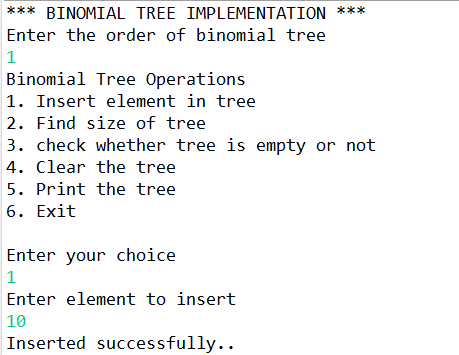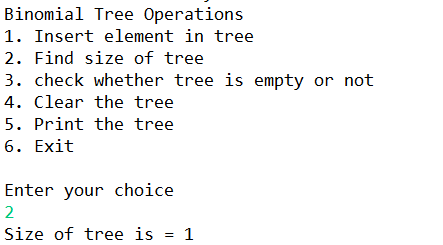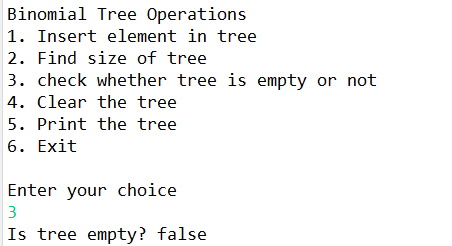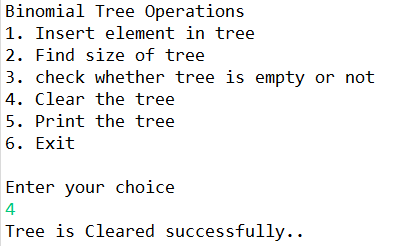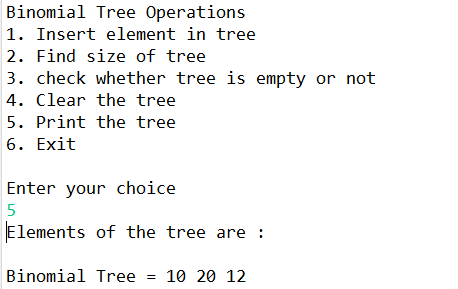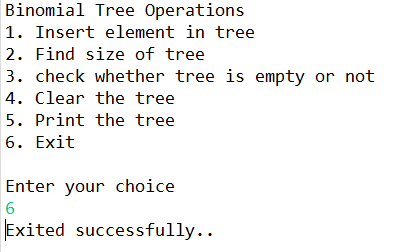Attention reader! Don’t stop learning now. Get hold of all the important Java Foundation and Collections concepts with the Fundamentals of Java and Java Collections Course at a student-friendly price and become industry ready. To complete your preparation from learning a language to DS Algo and many more,  please refer Complete Interview Preparation Course.

My Personal Notes arrow_drop_up